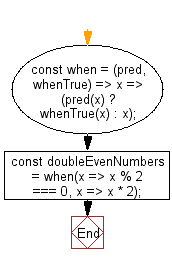# JavaScript: Test a value, x, against a predicate function

## JavaScript fundamental (ES6 Syntax): Exercise-104 with Solution

Write a JavaScript program to test a value, x, against a predicate function. If true, return fn(x). Else, return x.

Return a function expecting a single value, x, that returns the appropriate value based on pred.

• Return a function expecting a single value, x, that returns the appropriate value based on pred.

Sample Solution:

JavaScript Code:

``````//#Source https://bit.ly/2neWfJ2
const when = (pred, whenTrue) => x => (pred(x) ? whenTrue(x) : x);
const doubleEvenNumbers = when(x => x % 2 === 0, x => x * 2);
console.log(doubleEvenNumbers(2));
console.log(doubleEvenNumbers(1));
```
```

Sample Output:

```4
1
```

Pictorial Presentation:Flowchart:Live Demo:

See the Pen javascript-basic-exercise-104-1 by w3resource (@w3resource) on CodePen.

Improve this sample solution and post your code through Disqus

What is the difficulty level of this exercise?

Test your Programming skills with w3resource's quiz.

﻿